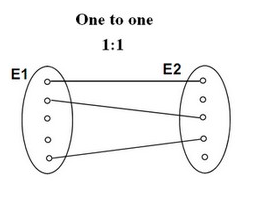# Function - Binary Function/Operation

A binary operation is an scalar operation with two arguments (arity of two) that produces one value.

They are creating a binary relation.

## List

$$f(x,y) = 2x.y$$

## Implementation

Discover MoreFunction - Arity

The number of argument in a function signature is called the arity 0-ary - - 0 argument 1-ary - - 1 argument 2-ary - - two arguments 3-ary - Ternary - three arguments. Example: three-valued...Function - Scalar

Scalar functions return a single value (as opposed to an array). Mathematics definition: Any function whose domain is a vector space and whose value is its scalar field. Is the result of a scalar function...Logical Data Modeling - Binary Relation

A binary relation is a relationship between two elements that is implemented via a binary function. Binary relations are used in many branches of mathematics to model concepts like: order relation...Mathematics - Exponentiation (square, cube) - Power

Exponentiation is a binary operation involving two numbers: the base (b) the exponent (n) (or index or power). In text notation or computer language, generally the exponentiation operator...Number - Addition (or Sum, Sigma) or Total

The addition of all numbers in a set is called a total. Sum can be use as: an aggregate or a analytic function. It can follow the full syntax of the analytic function and in this way create It's...Number - Division

A division is a mathematical binary function between 2 numbers. It is the definition of every rational number. where: The division symbol ÷ is just a blank fraction with dots replacing...Number - Multiplication (Product)

Multiplication is denoted by: the cross symbol × (\times in Latex), by a point · or by the absence of symbol It's a binary function. Multiplication is: Associative: By: Rafael...Set Theory - Bijection function (One-to-one relationship)

A Bijection is a mathematical / set function between the elements of two sets (ie binary function) where each element of one set is paired with exactly one element of the other set, each element...